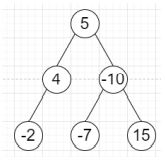# Program to traverse binary tree level wise in alternating way in Python

Suppose we have binary tree, we have to show the values of each level by alternating from going left-to-right and right-to-left.

So, if the input is likethen the output will be [5, -10, 4, -2, -7, 15]

To solve this, we will follow these steps −

• if root is null, then

• return a new list

• s1 := a list initially insert root

• s2 := a new list

• res := a new list

• while s1 is not empty or s2 is not empty, do

• while s1 is not empty, do

• node := delete last element from s1

• if left of node is not null, then

• insert left of node at the end of s2

• if right of node is not null, then

• insert right of node at the end of s2

• insert value of node at the end of res

• while s2 is not empty, do

• node := delete last element from s2

• if right of node is not null, then

• insert right of node at the end of s1

• if left of node is not empty, then

• insert left of node at the end of s1

• insert value of node at the end of res

• return res

Let us see the following implementation to get better understanding −

## Example

Live Demo

class TreeNode:
def __init__(self, data, left = None, right = None):
self.val = data
self.left = left
self.right = right

class Solution:
def solve(self, root):
if not root:
return []
s1 = [root]
s2 = []
res = []
while s1 or s2:
while s1:
node = s1.pop()
if node.left:
s2.append(node.left)
if node.right:
s2.append(node.right)
res.append(node.val)
while s2:
node = s2.pop()
if node.right:
s1.append(node.right)
if node.left:
s1.append(node.left)
res.append(node.val)
return res

ob = Solution()
root = TreeNode(5)
root.left = TreeNode(4)
root.right = TreeNode(-10)
root.left.left = TreeNode(-2)
root.right.left = TreeNode(-7)
root.right.right = TreeNode(15)
print(ob.solve(root))

## Input

root = TreeNode(5)
root.left = TreeNode(4)
root.right = TreeNode(-10)
root.left.left = TreeNode(-2)
root.right.left = TreeNode(-7)
root.right.right = TreeNode(15)

## Output

[5, -10, 4, -2, -7, 15]